Quasi-Harmonic Approximation¶

The following tutorial builds upon the earlier Lattice Dynamics tutorial. The information will not be repeated here.

Note: The QHA calculations are time consuming and precision-sensitive the calculations below present only the scheme of the procedure and may not be taken as an example of production runs. To obtain any valuable results you need to take special care to the quality of the phonon calculations involved and inspect all intermediary results.

The calculation requires the same setup as lattice dynamics tutorial but is inherently time-consuming. It is best done using a multi-core machine (perhaps in some computing center). The initialization part is the same as in other tutorials (cell [1-3])

In :
# Import the basic libraries
from __future__ import division
# ASE system
import ase
from ase import Atom, Atoms
from ase import io
from ase.lattice.spacegroup import crystal

# Spacegroup/symmetry library
from pyspglib import spglib

# Import the remote execution tools from the qe-util package
from qeutil import RemoteQE

# Utility function for plotting standard phonon plots
from qeutil.analyzers import plot_phonons, get_thermodynamic_functions, analyze_QHA_run, fit_and_plot_QHA

Calculator setup¶

This is the same setup as for the lattice dynamics calculation. Note, again, that the parameters here are selected not for the accuracy but for speed - the consequences of which will be evident in the final results.

In :
# Import actual access info for the remote execution from the external file.
import host
In :
# Create a Quantum Espresso calculator for our work.
# This object encapsulates all parameters of the calculation,
# not the system we are investigating.
qe=RemoteQE(label='SiC-QHA',
kpts=[4,4,4],    # Sampling grid in the reciprocal space for the electronic calculation
qpts=[4,4,4],    # Sampling grid in the reciprocal space for the phonon calculation
xc='pz',         # Exchange functional type in the name of the pseudopotentials
pp_type='vbc',   # Variant of the pseudopotential
pp_format='UPF', # Format of the pseudopotential files
ecutwfc=70,
pseudo_dir='../../pspot',
use_symmetry=True,
procs=8)         # Use 8 cores for the calculation

# Setup parameters for the second and third step of the procedure as well as for plotting.
# Since we will use multiple copies of the qe calculator we need to set up all parameters in advance
# Several special points in the FCC lattice for conventional dispersion curves plot
G1=[0,0,0]
G2=[1,1,1]
L=[1/2,1/2,1/2]
X1=[1,0,0]
K=[sqrt(1/2),sqrt(1/2),0]
X2=[1,1,0]

# Set the path along which we would like to plot the phonon dispersion curves
qpath=array([G1,X2,G2,L])
# Name the special points for plotting
qpname=[u'Γ','X',u'Γ','L']

# Put the parameters into the calculator
qe.set(qpath=qpath)       #   The path in the brillouin zone
qe.set(points=300);       #   Number of plot points between the special points along the dispersion curves
qe.set(nkdos=[15,15,15])      # Sampling grid in the reciprocal space for the PDOS integration
qe.set(ndos=200);              # Number of data points alog dos curve

# Check where the calculation files will reside on the local machine.
print qe.directory
calc/SiC-QHA.6j3CTa

Crystal setup¶

We use again a cubic SiC crystal. The setup of the crystal is the same as usual.

In :
# Stup the SiC crystal
# Create a cubic crystal with a spacegroup F-43m (216)
a=4.36
SiC = crystal(['Si', 'C'],
[(0, 0, 0), (0.25, 0.25, 0.25)],
spacegroup=216,
cellpar=[a, a, a, 90, 90, 90])

# Assign the calculator to our system
SiC.set_calculator(qe)

Calculation procedure¶

The essentials of the Quasi-Harmonic Approximations are described in details in many papers (e.g. P. Piekarz, et. al; J. Chem. Phys. 117 (2002) 3340). Here we just describe the practical routine. This type of calculation (similarly to the elastic constants calculation) is particularly well suited for automatization - since it involves many similar calculations which can be executed in parallel and analysed automatically.

The steps we need to take are:

1. Calculate the phonon dispersion for the base structure and verify the quality of the results (this has been done in the lattice dynamics tutorial. Thus, there is no need to repeat it here, since we use the same calculation parameters).
2. Ganerate a series of structures with the appropriate range of volumes. Depending on the thermal expansivity of the material this range may vary but for hard materials the range (99% - 102%) is usually sufficient. It may not be appropriate for other materials. Generally the higher the thermal expansivity, the wider should be the interval - with regard to the upper bound.
3. For each structure (volume) the system must be fully relaxed (internal degrees of freedom and the cell shape - with constant cell volume). This step was covered in the "Structural optimization" tutorial, and will not be repeated here. Furthermore, for the high-symmetry case of the Zinc-blende cubic crystal this step is actually unnecessary (there are no degrees of freedom to relax).
4. At each volume point the full phonon calculation should be performed. In principle, only the phonon-DOS function is required. Nevertheless, you should calculate and inspect phonon dispersion for each volume point to spot any problems with the results (soft modes, anomalies, etc.). The additional computation cost is minimal - the most time consuming step is the d2 calculation (second derivatives of energy).
5. For each temperature in the desired range calculate the phononic contribution to the Gibbs free energy and add it to the rest of the components.
6. Find the minimum of the Gibbs free energy at each temperature. The procedures in the qeutil.analyzers module use the Birch-Murnaghan logarithmic equation of state for the fitting procedure. It is possible to change the type of the fitted function. You can provide your own fitting function as an eos_fun argument to the fit_and_plot_QHA function. The function must be similar to:
def eos_fun(v,p):
return function(v,p,p....)

Note however that unless the p and p parameters of your function are minimum energy and volume of the minimum, respectively, the plotting function of the fit_and_plot_QHA procedure will not work properly. You will also need to re-process the output array from this procedure to extract any information it contains in the fitted parameters.
7. Process the set of fitted parameters to extract the thermal expansion curve as well as other parameters such as thermal expansivity or thermal dependence of bulk modulus. You can further use the obtained thermal expansion curve to generate a temperature scan (series of volumes corresponding to the grid of temperatures) and calculating other properties (e.g. elastic constants - see the elastic constants tutorial) for these structures - which then may be plotted as a function of temperature.

Volume scan¶

At this stage we should calculate the phonons for the basic structure. But since we have done that already in the "Lattice dynamics" tutorial we skip directly to the generation of the volume scan.

In :
# Generate a series of structures with volumes around basic equilibrium structure
calclst=[]                                           # Storage for structures to be calculated
for x in linspace(0.99,1.02,10):                     # Loop over the range of scalling factors
a=Atoms(SiC)                                     # Copy the basic structure
a.set_cell(SiC.get_cell()*x,scale_atoms=True)    # Scale the unit cell by x
a.set_calculator(qe.copy())                      # Assign the copy of the calculator
calclst.append(a)                                # Store the crystal

The calclst list contains the target series of structures. At this point - if our structures would be lower symmetry (i.e. contained any additional degrees of freedom except volume) we would need to run the structural optimization for each of them. Since the Zinc-blende crystal structure does not have any additional degrees of freedom we pass directly to phonon calculation. We run all of the structures in parallel to speed-up the process. This is a standard lattice dynamics calculation described in the previous tutorial. Nevertheless, you should pay special attention to the quality of the PDOS function. It should be calculated over a dense mesh with large number of points (ndos parameter) forming the DOS function.

In :
# Run the first two stages of the task in parallel
qe.ParallelCalculate(calclst,properties=['energy','d2']);
Launching: 1 2 3 4 5 6 7 8 9 10
Done: 1 2 3 4 5 6 7 8 9 10
In :
# Phonon dos and dispersion relations
qe.ParallelCalculate(calclst,properties=['phdos','frequencies']);
Launching: 1 2 3 4 5 6 7 8 9 10
Done: 1 2 3 4 5 6 7 8 9 10

Collection and analysis of the data¶

The results of each calculation are stored in the calc.results dictionary of each calculated structure. At this point we should review the quality of the obtained phonon data. As an absolute minimum we need to plot the PDOS curves to spot any irregularities or negative frequencies etc. Each suspected case should be investigated. The quality of further results critically depends on the quality of the obtained phonon modes.

In :
# Plot the calculated PDOS curves
# and report on the calculation directories to enable the manual inspection of the calculation
figsize(9,5)
for c in calclst:
r=c.calc.results
print "Lattice factor: %.3f" % ((c.get_volume()/SiC.get_volume())**(1.0/3),),
print " Directory:", c.calc.directory
plot(r['phdos'], r['phdos'],
label='x=%.3f ; %s' % ((c.get_volume()/SiC.get_volume())**(1.0/3),c.calc.directory))
xlabel('Frequency (cm$^{-1}$)')
ylabel('Phonon DOS (a.u.)')
legend(loc='upper left');
Lattice factor: 0.990  Directory: calc/SiC-QHA.zx_F3f
Lattice factor: 0.993  Directory: calc/SiC-QHA.7JQ8AT
Lattice factor: 0.997  Directory: calc/SiC-QHA.wBsZkg
Lattice factor: 1.000  Directory: calc/SiC-QHA.BcGgQm
Lattice factor: 1.003  Directory: calc/SiC-QHA.mQVLJV
Lattice factor: 1.007  Directory: calc/SiC-QHA.9fXpsL
Lattice factor: 1.010  Directory: calc/SiC-QHA.UOQLsx
Lattice factor: 1.013  Directory: calc/SiC-QHA.BAtQB9
Lattice factor: 1.020  Directory: calc/SiC-QHA.olcNVY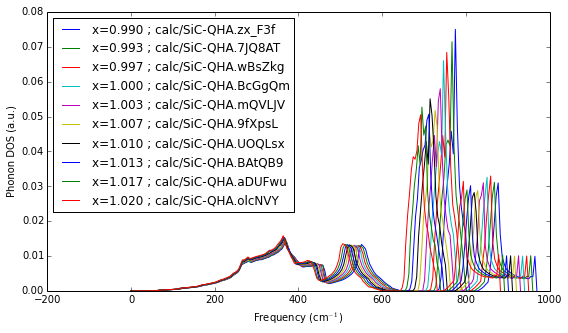If we are satisfied with the PDOS data we can proceed to the main QHA analysis. The qeutil.analyzers module provides two functions designed to help with this task.

• analyze_QHA_run which takes the list of calculations and calculates the Gibbs free energy for the Tsteps temperature steps in the temperature range (Tmin, Tmax). The output is an array of useful parameters calculated at each temperature point for each structure. At present the array contains: $[V$, $T$, $E_{tot}$, $F_{phonon}$, $P*V$, $C_V$, $S]$. The list may be expanded in the future.

• fit_and_plot_QHA is basically an implementation of the sixth point from the list above - given the array output by the analyze_QHA_run function it fits the equation of state function to the data and outputs the fitted parameters as a function of temperature. Additionally the function provides the plot of nop selected temperature points - to help in judging the quality of the data.

In :
# Calculate thermodynamic parameters as a function of temperature.
qha=analyze_QHA_run(calclst)
# 0: V=20.11 A^3, Etot=-263.130 eV, P=   3.3 GPa
# 1: V=20.31 A^3, Etot=-263.136 eV, P=   0.9 GPa
# 2: V=20.51 A^3, Etot=-263.137 eV, P=  -1.4 GPa
# 3: V=20.72 A^3, Etot=-263.135 eV, P=  -3.5 GPa
# 4: V=20.93 A^3, Etot=-263.132 eV, P=  -5.6 GPa
# 5: V=21.14 A^3, Etot=-263.125 eV, P=  -7.6 GPa
# 6: V=21.35 A^3, Etot=-263.116 eV, P=  -9.5 GPa
# 7: V=21.56 A^3, Etot=-263.104 eV, P= -11.4 GPa
# 8: V=21.77 A^3, Etot=-263.090 eV, P= -13.1 GPa
# 9: V=21.99 A^3, Etot=-263.073 eV, P= -14.8 GPa
/home/jochym/nipy/local/lib/python2.7/site-packages/qeutil/analyzers.py:293: RuntimeWarning: overflow encountered in square
Cv=ndf*k_B*simps(e*e*dos/sinh(e)**2,nu)
/home/jochym/nipy/local/lib/python2.7/site-packages/qeutil/analyzers.py:294: RuntimeWarning: overflow encountered in exp
S=k_B*(simps(2*dos*e/(exp(2*e)-1),nu)-simps(dos*(1-exp(-2*e)),nu))
In :
# Fit EOS to data and plot the results
figsize(10,7)
thexp=fit_and_plot_QHA(qha,nop=20);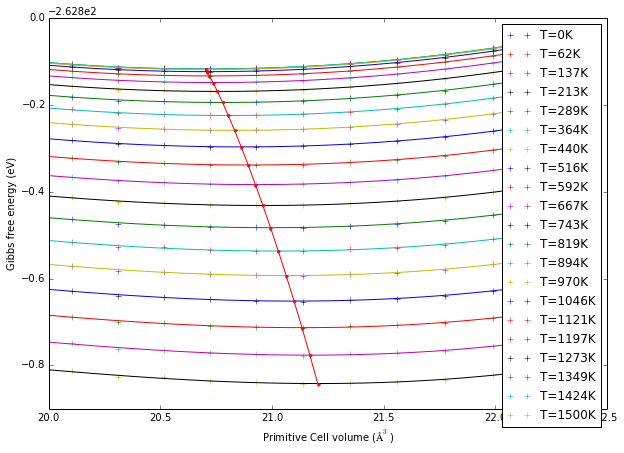Quality analysis¶

Even cursory review of the above data reveals some problems with our calculations. While the range of volumes seems adequate (maybe even too wide), the quality of the fits leaves something to be desired. At the typical scale of the plot it is hard to judge what is the reason for the high scatter of the points around the curves. To better assess the problem we will look closely at the low and high temperature data separately.

The closer examination reveals that both are actually problematic:

• The basic energy curve (low temperature) is evidently distorted in the low volume range - possibly an effect of too low energy cut-off for the calculation. This issue will mostly affect the low-temperature data.
• The high energy curve which is dominated by phonon contribution shows large scatter of this contribution - again, possibly due to the small reciprocal space sampling and too low quality of the PDOS function. Note that due to the sharp peaks present in the PDOS the integration procedures may be very sensitive to the quality of this function - you need to pay special attention to this issue. This problem will limit the range of temperatures we can investigate with our data and influence the quality of our high-temperature results.

There may be many other factors - you need to examine all possibilities to obtain meaningful results. But since this is a tutorial we will pass to the next stage to see what other data we may extract from the calculations and how the low quality of data points is reflected further down the line.

In :
# Select just the three first temperature points for plotting
fit_and_plot_QHA(qha[:,:3,:],nop=3);
show();
# And the last thee data points
fit_and_plot_QHA(qha[:,-3:,:],nop=3);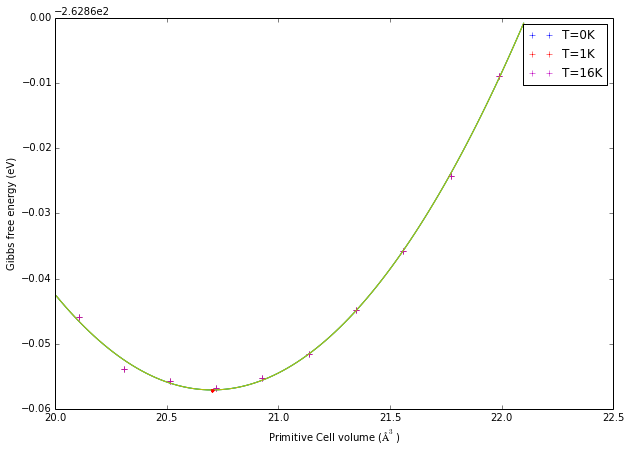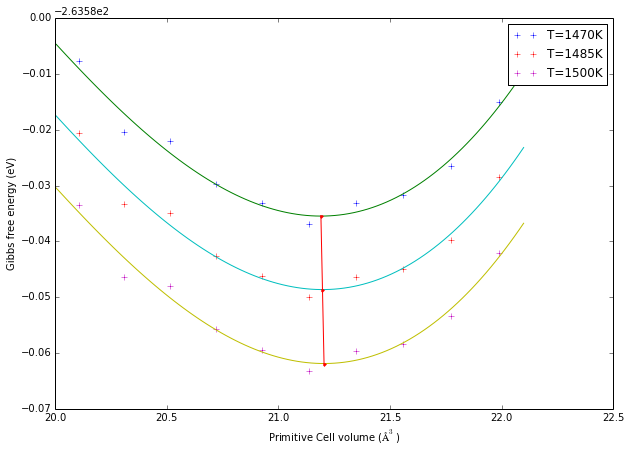Temperature-dependent properties¶

The fit_and_plot_QHA function returns the fit parameters as a function of temperature. The most important is the position of the minimum of free energy - which is our estimation of the equilibrium volume at each temperature. Plotted against the temperature it gives the thermal expansion curve. We can further derive the thermal expansion coefficient from this data.

Judging from the $\alpha$ curve in the inset we can decide that indeed the low-temperature data quality suffered badly due to the problems mentioned above.

In :
# Plot the thermal expansion
figsize(9,6)
plot(thexp,thexp);
xlabel('Temperature (K)')
ylabel('Volume ($\AA^3$)')
title('SiC thermal expansion')

# Calculate and plot the thermal expansion coefficient (alpha)
a = axes([.2, .5, .3, .3])                           # Create an inset plot
dt=thexp[0,2:]-thexp[0,:-2]                          # calculate the temperature steps
alpha=((thexp[1,2:]-thexp[1,:-2])/dt)/thexp[1,1:-1]  # calculate the derivative of volume with central formula
plot(thexp[0,1:-1],1e6*alpha);
title('Thermal expansion coeff.')
xlabel('Temperature (K)')
ylabel('$\\alpha_V * 10^6$');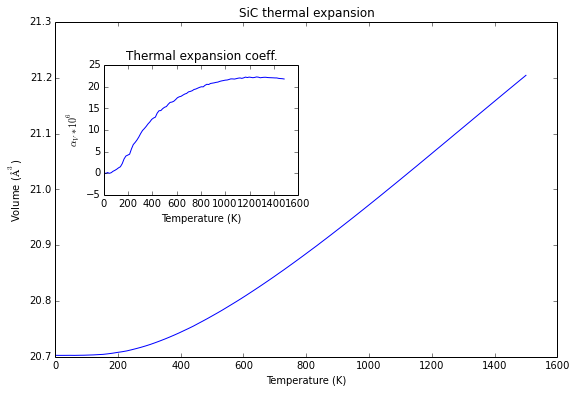Let us continue with the analysis of obtained data. The Birch-Murnaghan equation of state is parametrized by bulk modulus ($B_0$) and the temperature derivative ($B'_0$). Both quantities are included in the fit data obtained from the fit_and_plot_QHA function. Thus we have also thermal dependence of these two parameters.

In :
# Plot the bulk modulus as a function of temperature
plot(thexp,thexp/ase.units.GPa);
xlabel('Temperature (K)')
ylabel('$B_0$ (GPa)')
a = axes([.45, .55, .3, .3])                           # Create an inset plot
plot(thexp,thexp);
xlabel('Temperature (K)')
ylabel("$B_0'$");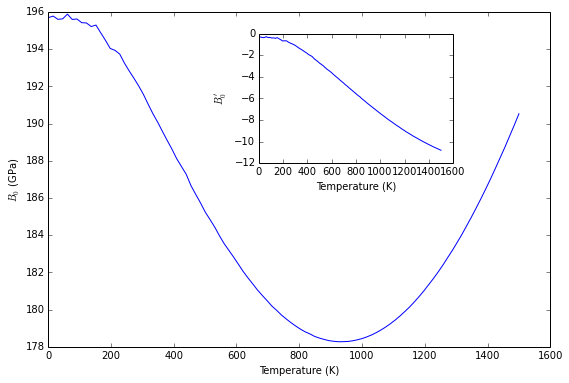It is quite clear that the low quality of the data points impacted the bulk modulus data as well. It is also evident from the non-physical shape of the high-temperature $B_0$ curve that the approximation - at least for this dataset - breaks down around T=700-800K. This should be no surprise considering the quality of the high-temperature data points and mentioned high sensitivity of the procedure to the accuracy of the data.